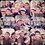# Cubic Number Pattern

Hey guys, I just want to share this simple things. I have found pattern of cubic number, and now I want to share it to you.

$$0^{3} = 0$$

$1^{3} = 1$

$2^{3} = 8 = 3 + 5$

$3^{3} = 27 = 7 + 9 + 11$

$4^{3} = 64 = 13 + 15 + 17 + 19$

$5^{3} = 125 = 21 + 23 + 25 +27 + 29$

$6^{3} = 216 = 31 + 33 + 35 + 37 + 39 + 41$

$7^{3} = 343 = 43 + 45 + 47 + 49 +51 + 53 + 55$

$8^{3} = 512 = 57 + 59 + 61 + 63 + 65 + 67 + 69 + 71$

$9^{3} = 729 = 73 + 75 + 77 + 79 + 81 + 83 + 85 + 87+ 89$

and so on and on and on !

Do you get the pattern ? Okay, any comments will be appreciated. Thank you...Note by Jonathan Christianto
5 years, 6 months ago

This discussion board is a place to discuss our Daily Challenges and the math and science related to those challenges. Explanations are more than just a solution — they should explain the steps and thinking strategies that you used to obtain the solution. Comments should further the discussion of math and science.

When posting on Brilliant:

• Use the emojis to react to an explanation, whether you're congratulating a job well done , or just really confused .
• Ask specific questions about the challenge or the steps in somebody's explanation. Well-posed questions can add a lot to the discussion, but posting "I don't understand!" doesn't help anyone.
• Try to contribute something new to the discussion, whether it is an extension, generalization or other idea related to the challenge.

MarkdownAppears as
*italics* or _italics_ italics
**bold** or __bold__ bold
- bulleted- list
• bulleted
• list
1. numbered2. list
1. numbered
2. list
Note: you must add a full line of space before and after lists for them to show up correctly
paragraph 1paragraph 2

paragraph 1

paragraph 2

[example link](https://brilliant.org)example link
> This is a quote
This is a quote
    # I indented these lines
# 4 spaces, and now they show
# up as a code block.

print "hello world"
# I indented these lines
# 4 spaces, and now they show
# up as a code block.

print "hello world"
MathAppears as
Remember to wrap math in $$ ... $$ or $ ... $ to ensure proper formatting.
2 \times 3 $2 \times 3$
2^{34} $2^{34}$
a_{i-1} $a_{i-1}$
\frac{2}{3} $\frac{2}{3}$
\sqrt{2} $\sqrt{2}$
\sum_{i=1}^3 $\sum_{i=1}^3$
\sin \theta $\sin \theta$
\boxed{123} $\boxed{123}$

Sort by:

Let me give you a pattern:

$1^{3} = 1^{2} = 1$

$1^{3}+2^{3} = (1+2)^{2} = 9$

$1^{3}+2^{3}+3^{3} = (1+2+3)^{2} = 36$

$1^{3}+2^{3}+3^{3}+4^{3} = (1+2+3+4)^{2} = 100$

In general,

$\boxed{1^{3}+2^{3}+\cdots+n^{3} = (1+2+\cdots+n)^{2}}$

Can you figure out why this is? ;)

- 5 years, 6 months ago

It should be $1^3=1^2=1$ in first row to make it (look like) a pattern. $\ddot\smile$

- 5 years, 6 months ago

Indeed it should, thanks for spotting that!

- 5 years, 6 months ago

In boxed part, $1^3+2^3$

- 5 years, 6 months ago

- 5 years, 6 months ago

I have edited it! $\ddot\smile$

- 5 years, 6 months ago

Sum of cubes = n(n+1)(n)(n+1)/4= [n(n+1)/2]^2= [Sum of the numbers]^2

- 5 years, 6 months ago

$\displaystyle \sum _{ k=0 }^{ n-1 }{ \left( { n }^{ 2 }-n+1+2k \right) ={ n }^{ 3 } }$

The cube creeps into this thing because of that first term ${ n }^{ 2 }$

As an example, this is true also, but the sum are quartics.

$\displaystyle \sum _{ k=0 }^{ n-1 }{ \left( { n }^{ 3 }-n+1+2k \right) ={ n }^{ 4 } }$

In fact, for any $a$, this is true

$\displaystyle \sum _{ k=0 }^{ n-1 }{ \left( { n }^{ a }-n+1+2k \right) ={ n }^{ a+1 } }$

- 5 years, 6 months ago

Correct me if I am wrong. So, for $n^{2}$ it will be

$\sum_{k=0}^{n-1} (n+1+2k) = n^{2}$

- 5 years, 6 months ago

Actually, leave out $n$, since ${n}^{1}-n=0$, and we have the classic sum of odd numbers $1+2k$ to make squares.

- 5 years, 6 months ago

Thanks for formula for ANY power.

- 5 years, 2 months ago

General term

$(n^2 - n + 1) + (n^2 - n + 3) + ..... + (n^2 + n -1) = n^3$

- 5 years, 6 months ago

Can you give the example please ??

- 5 years, 6 months ago

what are you not able to understand , can you tell?

- 5 years, 6 months ago

@U Z You have given me the general term. Can you give the example ?? For example, the result of $3^{3}$ using the general term. Can you catch it ??

- 5 years, 6 months ago

$3^3=(3^2-3+1)+(3^2-3+3)+(3^2-3+5)\\=7+9+11\\=27$

- 5 years, 6 months ago

I got it now, thanks...

- 5 years, 6 months ago

By the way, a good pattern and the thing I observed is There is a difference of 2 between consecutive numbers

- 5 years, 6 months ago

Can you explain it to me ?? For additional information for me, hehehe...

- 5 years, 6 months ago

Odd number means difference of 2.

- 5 years, 2 months ago

The cube of an integer $n$ can be written as sum of $n$ consecutive odd numbers, first odd number being $(n-1)^2-n$.

- 5 years, 6 months ago

$\sum_{i=1}^{n} \left(n(n-1)-1+2i\right) = n\times n(n-1)-n+n(n+1) = n^3$

- 5 years, 6 months ago